# Bisect

Bisect

Imagine a lovely cake with delicious frosting that needs to be divided at a birthday. The person cutting the cake will not divide the cake into multiple pieces, as it will create quite the mess.Instead, the person will first divide the cake into two equal halves. This is called bisection and it is an important part of geometry and how we study angles.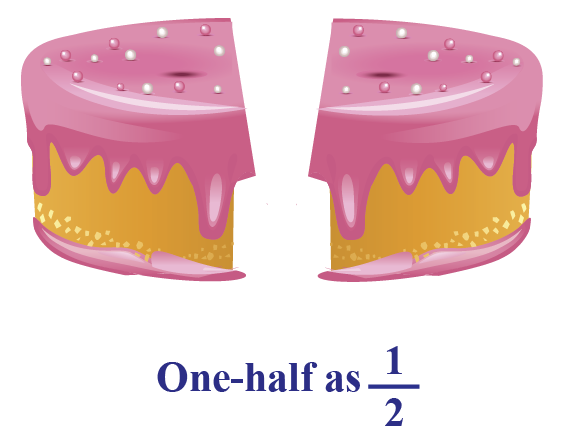In this lesson, we will learn how to bisect a segment, how to bisect lines, and the rules that are applied while bisecting angles.

Check out the interactive simulations to know more about the lesson and try your hand at solving a few interesting practice questions at the end of the page.

## Lesson Plan

 1 What is Bisect? 2 Thinking out of Box! 3 Important Notes on Bisect 4 Solved Examples on Bisect 5 Interactive Questions on Bisect

## What is Bisect?

### Bisect: Definition

Bisect means to cut or divide into two equal parts.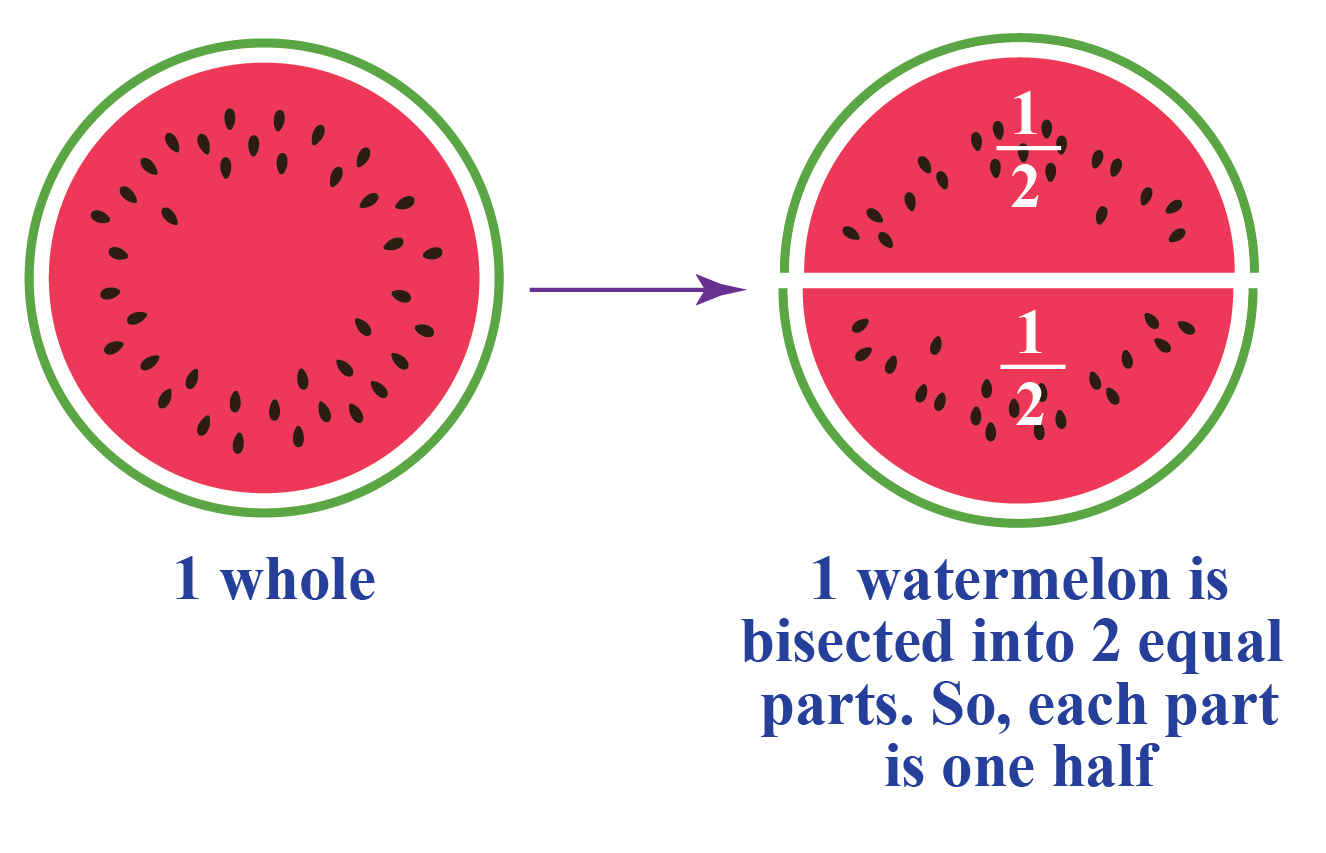## Bisecting a Line Segment

Let's do an activity to understand the meaning of bisecting a line segment.

Fold a sheet of paper and let $$\overline{AB}$$ be the line of fold.

Put an ink-dot on any one side of $$\overline{AB}$$ and name it as $$X$$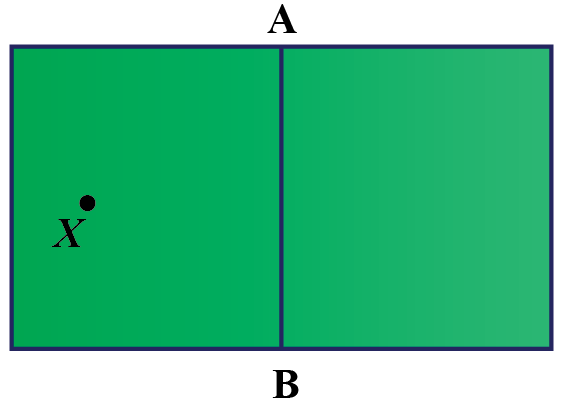Can you find the mirror image of $$X$$?

Name the mirror image of $$X$$ as $$X'$$.

Let $$\overline{AB}$$ and $$\overline{XX'}$$ intersect at $$O$$.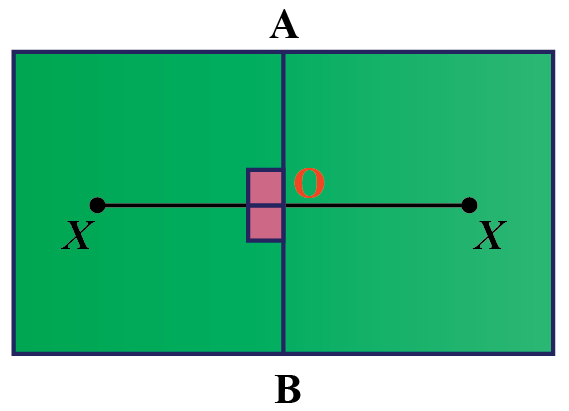Is $$\overline{OX}$$ and $$\overline{OX'}$$ equal?

This means $$\overline{AB}$$ divides $$\overline{XX'}$$ into two equal parts.

So, here $$\overline{AB}$$ bisects $$\overline{XX'}$$ and $$\overline{AB}$$ is a bisector of $$\overline{XX'}$$.

Use the simulation below to learn how to bisect a line segment using a ruler and a compass.

## Bisecting an Angle

Let's do another activity to understand how to bisect an angle.

Mark a point $$O$$ on a paper.

Taking $$O$$ as the initial point, draw two rays $$\overline{OA}$$ and $$\overline{OB}$$

You will get $$\angle AOB$$

Now, fold the sheet through $$O$$ such that the rays $$\overline{OA}$$ and $$\overline{OB}$$ overlap each other.

Name the line of the crease on the paper as $$\overline{OC}$$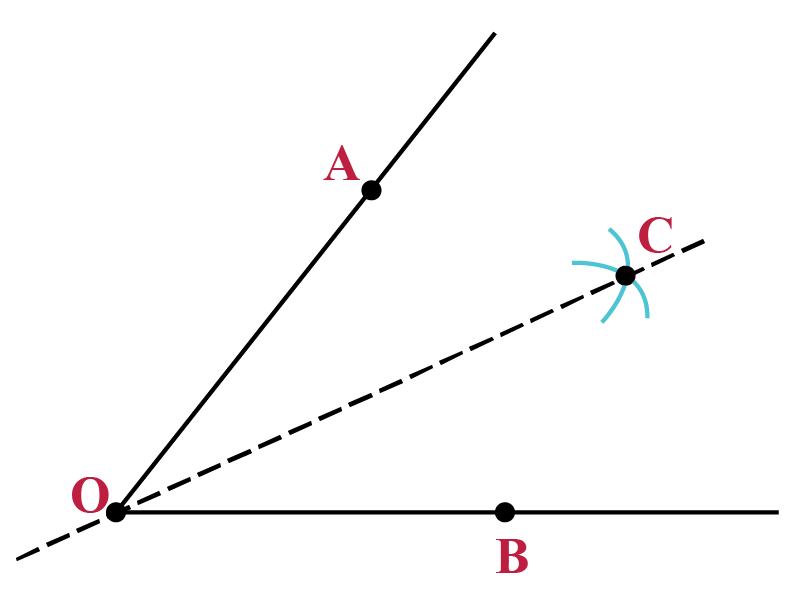Can you measure the angles $$\angle AOC$$ and $$\angle BOC$$? Are the measures equal?

Yes, both the angles are equal and $$OC$$ is the angle bisector of $$\angle AOB$$.

Use the simulation below to learn the way to bisect an angle using a ruler and a compass.Think Tank
1. A ray $$BD$$ bisects an angle $$\angle ABC$$. If $$\angle ABC=120^{\circ}$$, what would be the measure of the angles $$\angle ABD$$ and $$\angle DBC$$?
2. Find the coordinates of the midpoint of the line segment $$\overline{AB}$$ joining the points $$(-5,3)$$ and $$(3, 2)$$.

## Bisecting a Shape

Some shapes can also be bisected.

Look at the shapes shown below.

A line segment bisects each shape into two equal parts.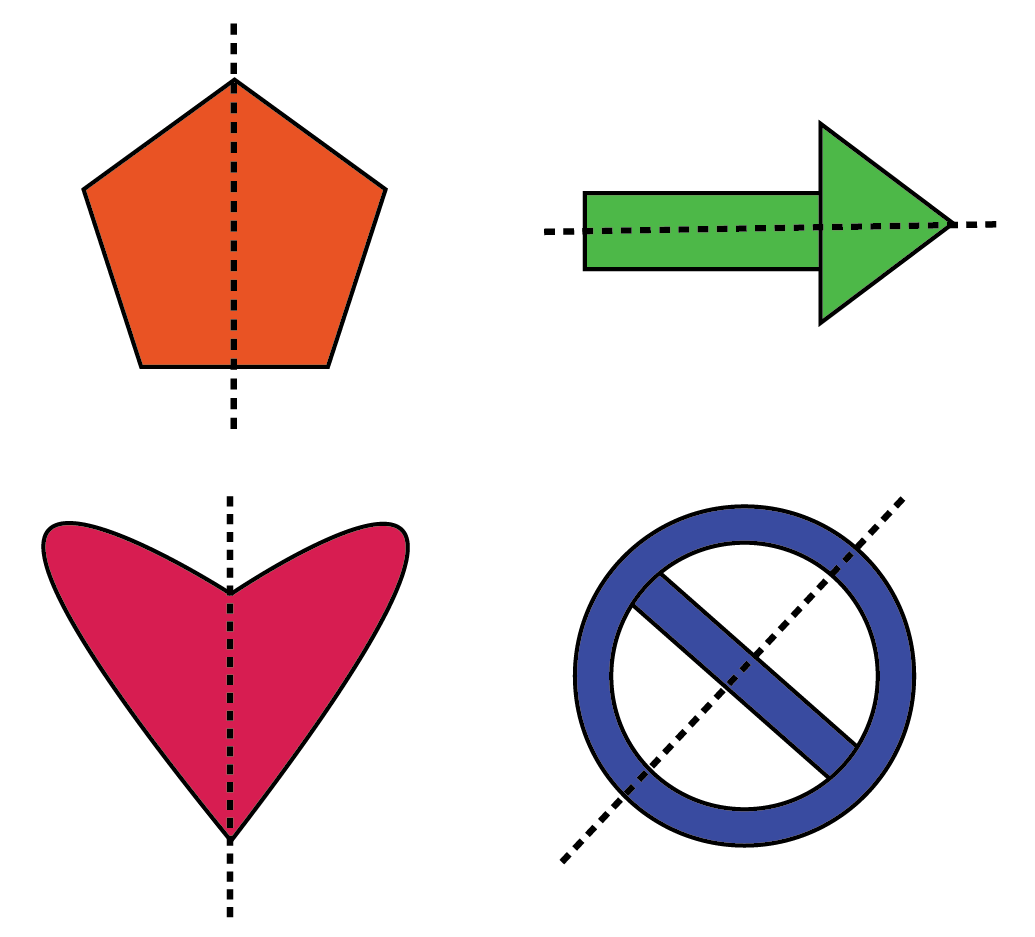More Important Topics
More Important Topics